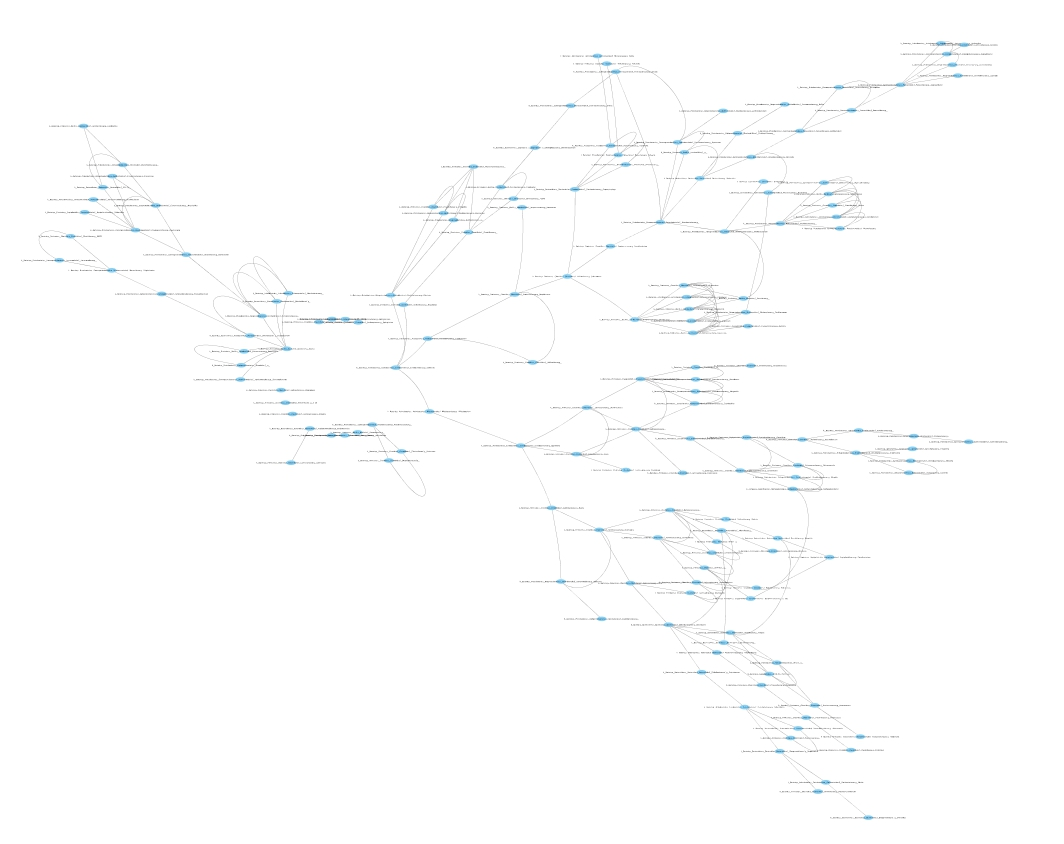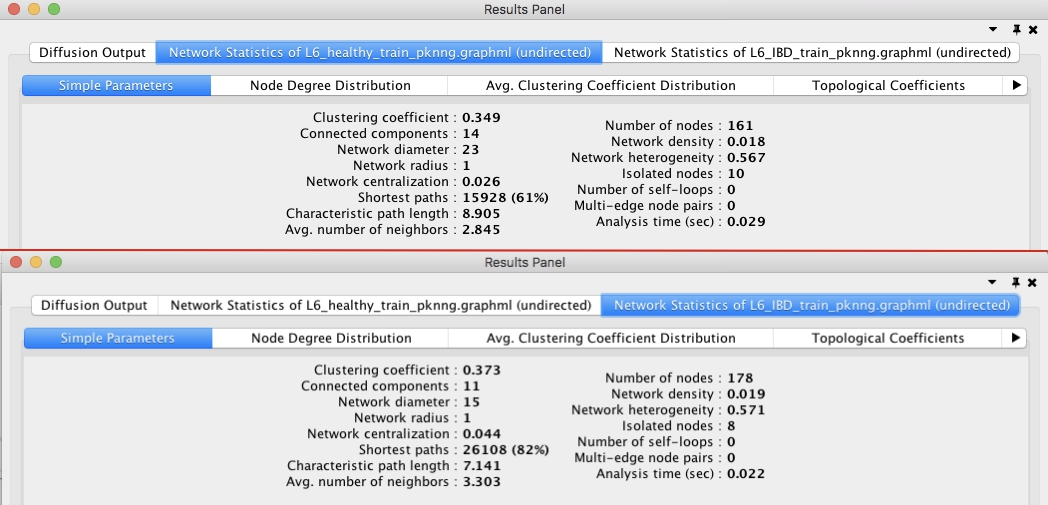Comparative network analysis of perturbed kNN graphs¶

by Yasser El-Manzalawy yasser@idsrlab.com

In this tutorial, we construct two perturbed kNN graph for IBD and healthy controls (respectively) and then present examples of possible comparative network analysis that could be apply to the two graphs using Cytoscape. In particular, we compare the two graphs using: - Their global topological properties obtained using Cytoscape NetworkAnalyzer tool - Their top modules obtained using MCODE plugins - Their most varying nodes using DyNet Analyzer plugins and we report the subnetwork of top most varying 20 nodes (potential IBD biomarkers)

In :
import numpy as np
import pandas as pd
import networkx as nx

from proxi.algorithms.pknng import get_pknn_graph
from proxi.utils.misc import save_graph, save_weighted_graph
from proxi.utils.process import *
from proxi.utils.distance import abs_correlation

import warnings
warnings.filterwarnings("ignore")

Construct an undirected pkNN graph using IBD OTU table¶

In :
# Input file(s)
ibd_file = './data/L6_IBD_train.txt'   # OTU table

# Ouput file(s)
ibd_graph_file = './graphs/L6_IBD_train_pknng.graphml'   # Output file for pkNN graph

# Parameters
num_neighbors = 5        # Number of neighbors, k, for kNN graphs
dist = abs_correlation   # distance function
T=100                    # No of iterations
c=0.6                    # control parameter for pknng algorithm
In :
# Load OTU Table
df = pd.read_csv(ibd_file, sep='\t')

# Proprocess OTU Table by deleting OTUs with less than 5% non-zero values
df = select_top_OTUs(df, get_non_zero_percentage, 0.05, 'OTU_ID')

# Construct kNN-graph
nodes, a,_ = get_pknn_graph(df, k=num_neighbors, metric=dist, T=T, c=c)

# Save the constructed graph in an edge list format
save_graph(a, nodes, ibd_graph_file)

Shape of original data is (178, 200)

Fig. 1 shows the constructed perturbed kNN graph from IBD samples.Figure 1: Perturbed kNN undirected proximity graph constructed from IBD OTU table using k=5, T=100, and c=0.6.

Fig. 2 shows the constructed perturbed kNN graph from healthy control samples. Note that we don’t need to construct this network since it has been generated in tutorial 2.Figure 2: Perturbed kNN undirected proximity graph constructed from healthy OTU table using k=5, T=100, and c=0.6 (See Example_2).

Now, we can use cytoscape and some of its plugins to compare the two graphs in Figures 1 and 2.

Analysis of global topological properties¶

First, we used Cytoscape NetworkAnalyzer tool (1) to get several global properties of each network. Fig. 3 shows that IBD network has higher average node degree, clustering coefficient, network centralization, and number of nodes.Figure 3: Global network properties for healthy (top) and IBD (bottom) networks.

Analysis of top first modules¶

Second, we used MCODE (2) to extract top modules from each network. Fig. 4 compare the top first module from healthy (top) and IBD (bottom) networks. For healthy network, the top module includes interactions between 4 different genera of Firmicutes and 2 different genera of Actionbacteria. For IBD network, the top module includes interactions among different genara belonging to Actionbacteria, Proteobacteria, Firmicutes, and Bacteriodetes phylum.Figure 4: Top module extracted from healthy (top) and IBD (bottom) networks.

Analysis of most varying nodes¶

Third, we used DyNet Analyzer (3) to compare the the networks in healthy and IBD states. The results are visualized in Fig. 5 where: green edges represent edges present only in healthy network; red edges represent edges present only in IBD network; and gray edges represent edges present in both networks. DyNet also associates a rewiring score with each node that quantifies the amount of change in the identity of the node interacting neighbors. We then ranked nodes by their DyNet score and generated a subnetwork of the top 20 nodes (See Fig. 6). Interestingly, 13 out of 20 nodes form a single connected module. In this module, two nodes corresponding to corynebacterium genera and Rhodocyclaceae family have the highest node degrees of 5 and 4 (respectively).Figure 5: DynNet Analyzer. Healthy (green) and IBD (red).Figure 6: Subnetwork of top 20 varying nodes determined using DyNet score.

References:

 Assenov, Yassen, et al. “Computing topological parameters of biological networks.” Bioinformatics 24.2 (2007): 282-284.

 Bader, Gary D., and Christopher WV Hogue. “An automated method for finding molecular complexes in large protein interaction networks.” BMC bioinformatics 4.1 (2003): 2.

 Goenawan, Ivan H., Kenneth Bryan, and David J. Lynn. “DyNet: visualization and analysis of dynamic molecular interaction networks.” Bioinformatics 32.17 (2016): 2713-2715.